# PySurvival Tutorial: Churn Modeling

### Using PySurvival to Model Churn

This article is the second installment in a four part series, which will include tutorials designed to demonstrate how to easily make the most of the package. You can also find these tutorials on the official website: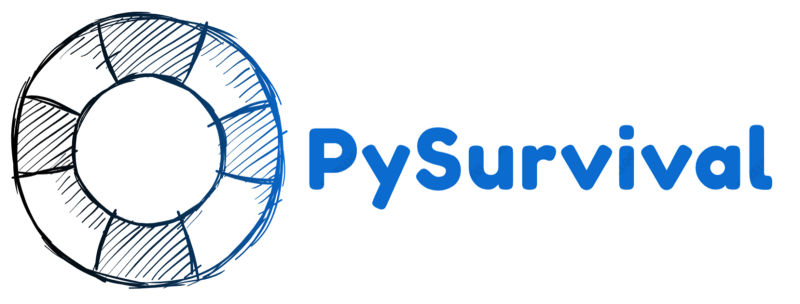## Introduction

Customer churn rate, or the percentage of customers that stop using a company’s products or services, is one of the most important metrics for a business, as it usually costs more to acquire new customers than it does to retain existing ones.

See, for instance, this study by Bain & Company, which suggests that existing customers tend to buy more from a company over time and may refer the products they use to others. For example, in financial services, a 5% increase in customer retention produces more than a 25% increase in profit.

With Survival Analysis, companies can better strategize around churn by predicting if and when customers are likely to stop doing business. Let’s look at an illustrative example.

## Background

A software as a service (SaaS) company provides a suite of products for small and medium-sized enterprises, such as data storage, accounting, travel and expense management as well as payroll management.

So as to help the company forecast the acquisition and marketing costs for the next fiscal year, the data science team wants to build a churn model to predict when customers are likely to stop their monthly subscription. Thus, once customers have been flagged as likely to churn within a certain time window, the company could take the necessary retention actions.

## Dataset

### Description and Overview

The description of the dataset the team wants to use can be found here. Let’s import the modules and load the dataset:

``````# Importing modules
import pandas as pd
import numpy as np
from matplotlib import pyplot as plt
from pysurvival.datasets import Dataset
%pylab inline

print("The raw_dataset has the following shape: {}.".format(raw_dataset.shape))
``````

Here is an overview of the raw dataset:

``````+--------------------+------------+--------+---------+
|product_data_storage| csat_score | ...    | churned |
|--------------------|------------|--------|---------|
| 1024               | 9          | ...    | 0       |
| 2048               | 10         | ...    | 0       |
``````

### From categorical to numerical

There are several categorical features that need to be encoded into one-hot vectors:

• `product_travel_expense`
• `product_payroll`
• `product_accounting`
• `us_region`
• `company_size`
``````# Creating one-hot vectors
categories = ['product_travel_expense', 'product_payroll', 'product_accounting',
'us_region', 'company_size']
dataset = pd.get_dummies(raw_dataset, columns=categories, drop_first=True)

# Creating the time and event columns
time_column = 'months_active'
event_column = 'churned'

# Extracting the features
features = np.setdiff1d(dataset.columns, [time_column, event_column] ).tolist()
``````

#### Exploratory Data Analysis

As this tutorial is mainly designed to provide an example of how to use PySurvival, we will not do a thorough exploratory data analysis here but greatly encourage the reader to do so by checking the predictive maintenance tutorial that provides a detailed analysis.

Here, we will just check if the dataset contains Null values or if it has duplicated rows. Then, we will take a look at feature correlations.

#### Null values and duplicates

The first thing to do is checking if the raw_dataset contains Null values and has duplicated rows.

``````# Checking for null values
N_null = sum(dataset[features].isnull().sum())
print("The raw_dataset contains {} null values".format(N_null)) #0 null values

# Removing duplicates if there exist
N_dupli = sum(dataset.duplicated(keep='first'))
dataset = dataset.drop_duplicates(keep='first').reset_index(drop=True)
print("The raw_dataset contains {} duplicates".format(N_dupli))

# Number of samples in the dataset
N = dataset.shape
``````

#### Correlations

Let’s compute and visualize the correlation between the features: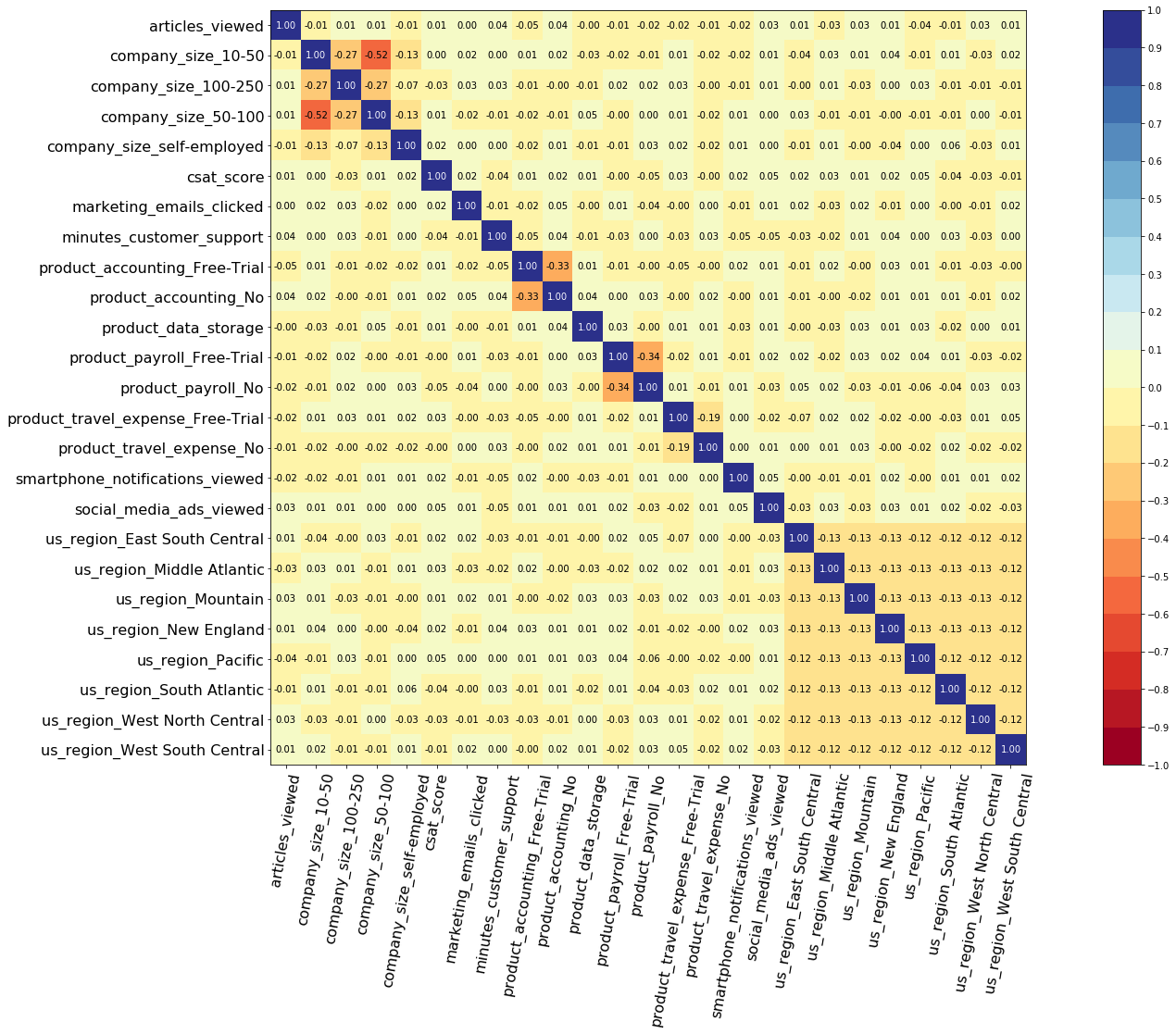As we can see, there aren’t any alarming correlations.

### Modeling

#### Building the model

To perform cross validation later on and assess the performance of the model, let’s split the dataset into training and testing sets.

``````# Building training and testing sets
from sklearn.model_selection import train_test_split
index_train, index_test = train_test_split( range(N), test_size = 0.35)
data_train = dataset.loc[index_train].reset_index( drop = True )
data_test  = dataset.loc[index_test].reset_index( drop = True )

# Creating the X, T and E inputs
X_train, X_test = data_train[features], data_test[features]
T_train, T_test = data_train[time_column], data_test[time_column]
E_train, E_test = data_train[event_column], data_test[event_column]
``````

Let’s now fit a Conditional Survival Forest model to the training set.

Note: The choice of the hyper-parameters was obtained using grid-search selection, not displayed in this tutorial.

``````from pysurvival.models.survival_forest import ConditionalSurvivalForestModel

# Fitting the model
csf = ConditionalSurvivalForestModel(num_trees=200)
csf.fit(X_train, T_train, E_train, max_features='sqrt',
max_depth=5, min_node_size=20, alpha=0.05, minprop=0.1)
``````

#### Variables importance

Having built a Survival Forest model allows us to compute the features importance:

``````# Computing variables importance
``````

Here are the top 5 of the most important features:

``````+----------------------------+-------------+----------------+
| feature                    | importance  | pct_importance |
|----------------------------|-------------|----------------|
| csat_score                 | 11.251287   | 0.176027       |
| product_payroll_No         | 11.204996   | 0.175303       |
| minutes_customer_support   | 9.167136    | 0.143421       |
| product_accounting_No      | 7.768278    | 0.121535       |
| product_payroll_Free-Trial | 3.669896    | 0.057416       |
+----------------------------+-------------+----------------+
``````

Thanks to the feature importance, we get a better understanding of what drives retention or churn. Here are the variables that play a critical role:

• accounting product usage

• payroll management product usage

• satisfaction survey score (a.k.a. csat)

• amount of time spent on the phone with customer support

Note: The importance is the difference in prediction error between the perturbed and unperturbed error rate as depicted by Breiman et al.

### Cross Validation

In order to assess the model performance, we previously split the original dataset into training and testing sets, so that we can now compute its performance metrics on the testing set:

#### C-index

The C-index represents the global assessment of the model discrimination power: this is the model’s ability to correctly provide a reliable ranking of the survival times based on the individual risk scores. In general, when the C-index is close to 1, the model has an almost perfect discriminatory power; but if it is close to 0.5, it has no ability to discriminate between low and high-risk subjects.

``````from pysurvival.utils.metrics import concordance_index
c_index = concordance_index(csf, X_test, T_test, E_test)
print('C-index: {:.2f}'.format(c_index)) #0.83
``````

#### Brier Score

The Brier score measures the average discrepancies between the status and the estimated probabilities at a given time. Thus, the lower the score (usually below 0.25), the better the predictive performance. To assess the overall error measure across multiple time points, the Integrated Brier Score (IBS) is usually computed as well.

``````from pysurvival.utils.display import integrated_brier_score
ibs = integrated_brier_score(csf, X_test, T_test, E_test, t_max=12,
figure_size=(15,5))
print('IBS: {:.2f}'.format(ibs))
``````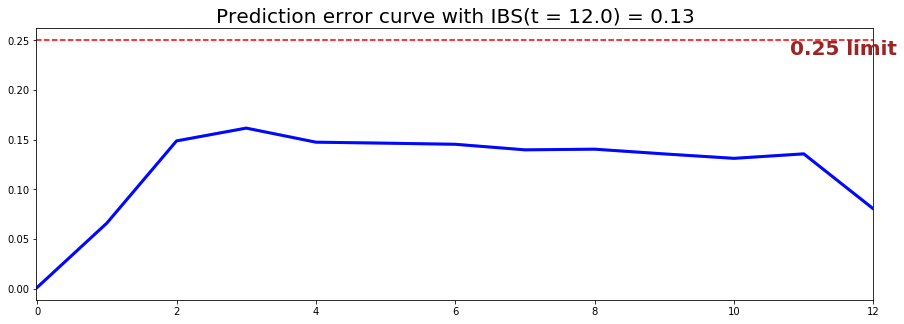The IBS is equal to 0.13 on the entire model time axis. This indicates that the model will have good predictive abilities.

Predictions

#### Overall predictions

Now that we have built a model that seems to provide great performances, let’s compare the time series of the actual and predicted number of customers who stop doing business with the SaaS company, for each time t.

``````from pysurvival.utils.display import compare_to_actual
results = compare_to_actual(csf, X_test, T_test, E_test,
is_at_risk = False,  figure_size=(16, 6),
metrics = ['rmse', 'mean', 'median'])
``````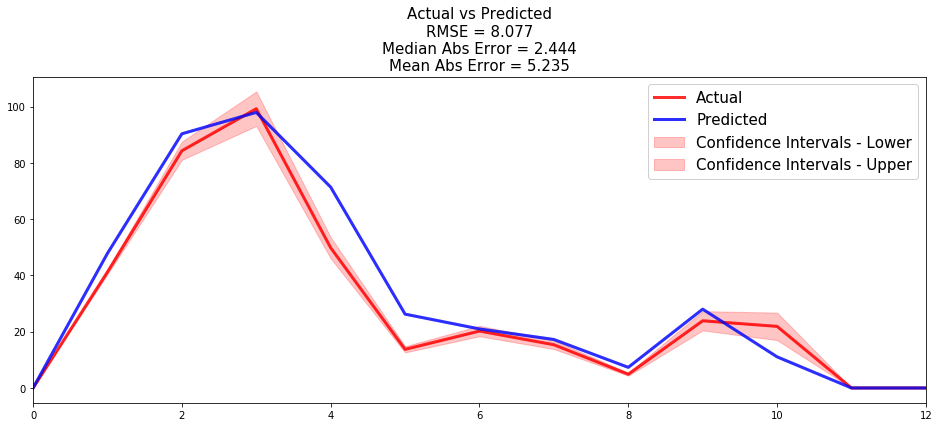The model provides very good results overall as on an entire 12 months window, it only makes an average absolute error of ~5 customers.

#### Individual predictions

Now that we know that we can provide reliable predictions for an entire cohort, let’s compute the probability of remaining a customer for all times t.

First, we can construct the risk groups based on risk scores distribution. The helper function `create_risk_groups`, which can be found in `pysurvival.utils.display`, will help us do that:

``````from pysurvival.utils.display import create_risk_groups

risk_groups = create_risk_groups(model=csf, X=X_test,
use_log = False, num_bins=30, figure_size=(20, 4),
low={'lower_bound':0, 'upper_bound':8.5, 'color':'red'},
medium={'lower_bound':8.5, 'upper_bound':12.,'color':'green'},
high={'lower_bound':12., 'upper_bound':25,  'color':'blue'}
)
``````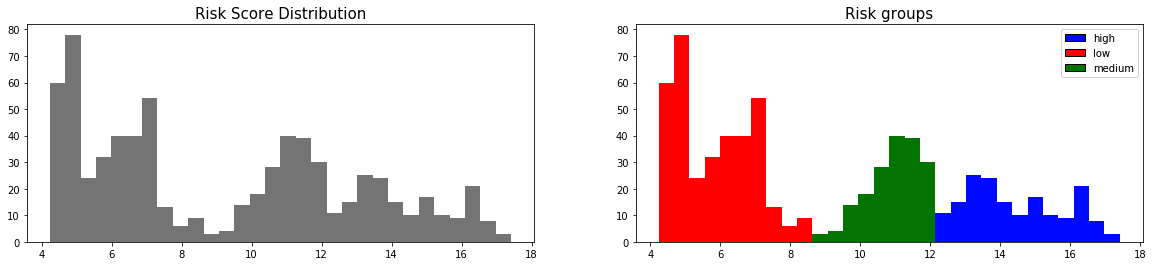Here, it is possible to distinguish 3 main groups: low, medium and high risk groups. Because the C-index is high, the model will be able to rank the survival times of a random unit of each group, such that: t_high ≤ t_medium≤ t_low

Let’s randomly select individual units in each group and compare their probability of remaining a customer for all times t. To demonstrate our point, we will purposely select units which experienced an event to visualize the actual time of event.

``````# Initializing the figure
fig, ax = plt.subplots(figsize=(15, 5))

# Selecting a random individual that experienced an event from each group
groups = []
for i, (label, (color, indexes)) in enumerate(risk_groups.items()) :

# Selecting the individuals that belong to this group
if len(indexes) == 0 :
continue
X = X_test.values[indexes, :]
T = T_test.values[indexes]
E = E_test.values[indexes]

# Randomly extracting an individual that experienced an event
choices = np.argwhere((E==1.)).flatten()
if len(choices) == 0 :
continue
k = np.random.choice( choices, 1)

# Saving the time of event
t = T[k]

# Computing the Survival function for all times t
survival = csf.predict_survival(X[k, :]).flatten()

# Displaying the functions
label_ = '{} risk'.format(label)
plt.plot(csf.times, survival, color = color, label=label_, lw=2)
groups.append(label)

# Actual time
plt.axvline(x=t, color=color, ls ='--')
ax.annotate('T={:.1f}'.format(t), xy=(t, 0.5*(1.+0.2*i)),
xytext=(t, 0.5*(1.+0.2*i)), fontsize=12)

# Show everything
groups_str = ', '.join(groups)
title = "Comparing Survival functions between {} risk grades".format(groups_str)
plt.legend(fontsize=12)
plt.title(title, fontsize=15)
plt.ylim(0, 1.05)
plt.show()
``````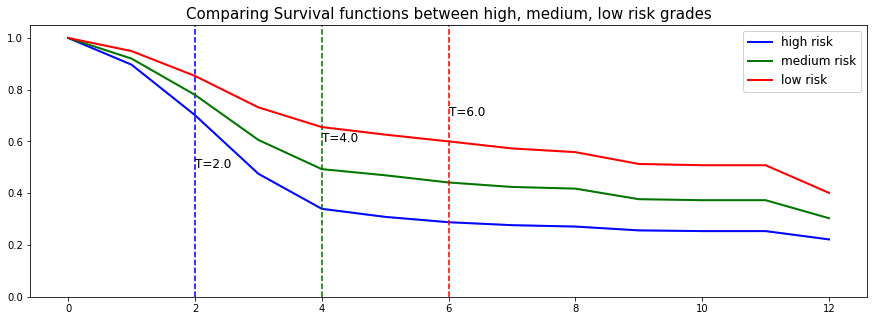Here we can see that the model manages to provide great prediction of the event time.

### Conclusion

We can now save our model so as to put it in production and score future customers.

``````# Let's now save our model
from pysurvival.utils import save_model
save_model(csf, '/Users/xxx/Desktop/churn_csf.zip')
``````

In conclusion, we can see that it is possible to predict when customers will stop doing business with the company at different time points. The model will help the company be more pro-active when it comes to retaining their customers; and provide a better understanding of the reasons that drive churn.

In the next article, we will dive into a complete tutorial on predictive maintenance.

For more information on the package, check out the official website here: https://www.pysurvival.io/